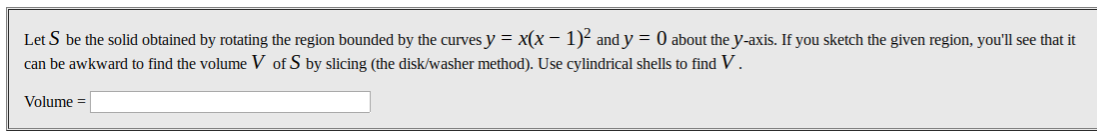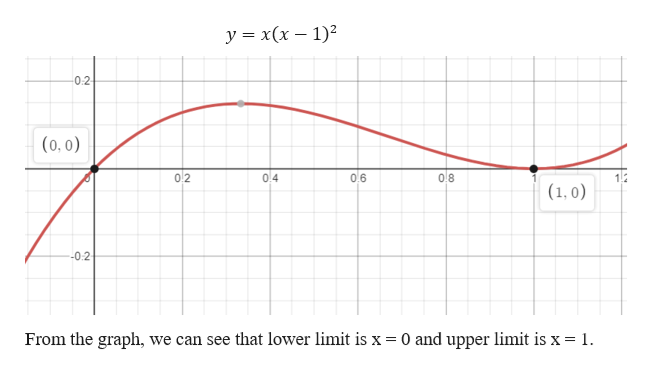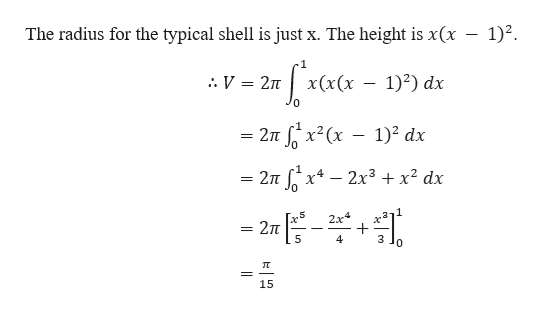# Let S be the solid obtained by rotating the region bounded by the curves y = x(x – 1)² and y = 0 about the y-axis. If you sketch the given region, you'll see that itcan be awkward to find the volume V of S by slicing (the disk/washer method). Use cylindrical shells to find V .Volume =

Question
68 viewshelp_outlineImage TranscriptioncloseLet S be the solid obtained by rotating the region bounded by the curves y = x(x – 1)² and y = 0 about the y-axis. If you sketch the given region, you'll see that it can be awkward to find the volume V of S by slicing (the disk/washer method). Use cylindrical shells to find V . Volume = fullscreen
check_circle

Step 1

Given,

Let S be the solid obtained by rotating the region bounded by the curves y = x (x – 1)² and y = 0 about the y-axis.

Graph of Curve is given below.help_outlineImage Transcriptioncloseу%3 х (х — 1)2 -02 (0, 0) 0.6 0.8 02 0.4 (1, 0) -0.2 From the graph, we can see that lower limit is x = 0 and upper limit is x = 1. fullscreen
Step 2

Now, using the cylindric...help_outlineImage TranscriptioncloseThe radius for the typical shell is just x. The height is x(x – 1)². : V = 2n x(x(x – 1)²) dx = 2n x?(x – 1)² dx 2n x* – 2x³ + x² dx 2x4 = 2n 4 3 15 fullscreen

### Want to see the full answer?

See Solution

#### Want to see this answer and more?

Solutions are written by subject experts who are available 24/7. Questions are typically answered within 1 hour.*

See Solution
*Response times may vary by subject and question.
Tagged in
MathCalculus

### Integration